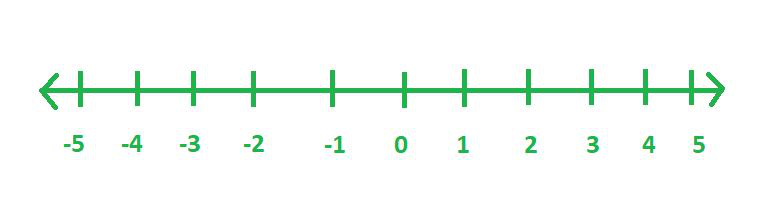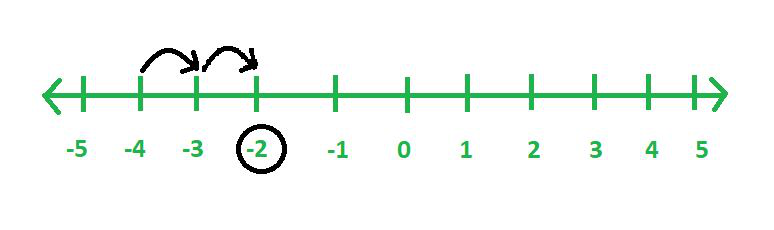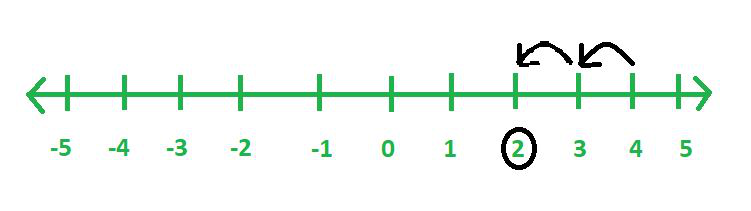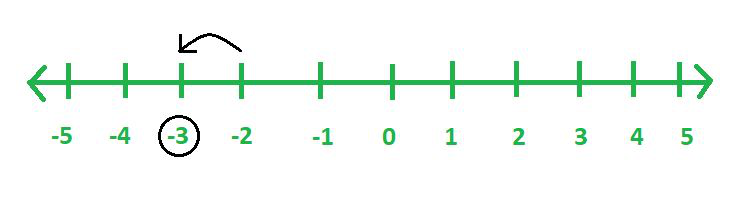GeeksforGeeks App
Open AppBrowser
Continue

# How to add the positive and negative integers?

Integers are a combination of whole numbers along with negative numbers. Thus, the numbers -3, -2, -1, 0, 1, 2, 3 are called integers.

• The natural numbers 1, 2, 3 are called positive integers.
• The negative numbers -3, -2, -1 are called negative integers.
• The number 0 is also an integer but it is neither a positive integer nor a negative integer.

Representation of integers on a Number Line

1. Draw a line and label a point on it as zero.
2. Select a unit of length. Start marking points on the line at unit length intervals on both sides of 0 (zero).
3. To the right side of the zero, label the points as 1, 2, 3, … and the points to the left of the zero as -1, -2, -3, …
4. Lastly, add arrows on either side of the line to show that the line continues indefinitely in both directions.### Addition of positive and negative integers using anumber line

• To add a positive number, we need to move to the right of the number line.
• To add a negative number, we need to move to the left of the number line.

### Sample Problems

Question 1: Find (-4) + 2.

Solution:

Start from -4 on the number line. Move 2 units to the right. We reach -2.

Therefore, (-4) + 2 = -2Question 2: Find 4 + (-2).

Solution:

Start from 4 on the number line. Move 2 units to the left. We reach +2.

Therefore, 4 + (-2) = 2Question 3: Find (-2) + (-1).

Solution:

Start from -2 on the number line. Move 1 unit to the left. We reach at -3.

Therefore, (-2) + (-1) = -3### Addition of positive and negative numbers without the number line

Using the number line to add large numbers is a very tedious task. There is a better alternative, we have the following rules for the addition of integers.

1. To add two positive numbers – add them as natural numbers.

2. To add two negative numbers – add their absolute values and finally give the sum a negative sign. While practising, do not consider the signs, find the sum of the numbers and give negative sign to the sum obtained.

3. To add a positive and negative number – subtract the smaller number from the larger number and give the sign of the number which is larger.

Example 1: Evaluate (+130) + (+200)

Solution:

As we can see here, both the numbers are positive, so we need to add them simply like natural numbers.

Therefore, (+130) + (+200) = 130 + 200 = 330.

Example 2: Evaluate (-130) + (-200)

Solution:

As we can see here, both the numbers are negative, so we need to add them simply as natural numbers and then give the sum a negative sign.

Therefore, (-130) + (-200) = -(130 + 200) = -330.

Adding One positive and one negative number

Example 3: Evaluate (-130) + (+200)

Solution:

As we can see here, one number is positive and another one is negative, so we need to subtract the smaller number from the larger number and give the sign of the larger number i.e. subtract 130 from 200 and give sign as positive to the final sum.

Therefore, (-130) + (+200) = 200 – 130 = +70.

My Personal Notes arrow_drop_up
Related Tutorials